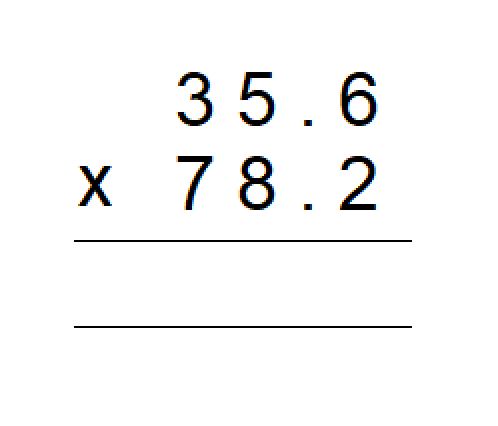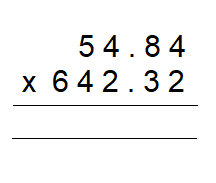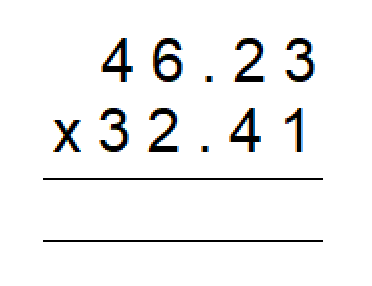# Understanding The Various Operations Of Decimals

In this quiz, different questions related to different operations of decimals are asked to clear the basic concept of the decimal to any student. This quiz includes various multiple-choice questions and various word problems are added in the quiz to let students understand the concept of decimals.

Start Quiz

245.24 + 23.23 = ##268.47##

268.47

74.54 + 83.12 = ##157.66##

157.66

Subtract the given decimals.

321.35 - 234.12 = ##87.23##

87.23

Subtract the given decimals.

235.23 - 163.34 = ##71.89##

71.89

Multiply.25253.3

27839.2

2783.92

2534.23

Multiply.35224.8288

46482.37

8383.2828

84732.2332

Multiply.7823.8723

2352.1342

8191.3233

1498.3143

Divide the given integers.

37.68 ÷ 3 = ##12.56##

12.56

Divide the given integers.

305.5 ÷ 5 = ##61.1##

61.1

Divide the given integers.

340.2 ÷ 4 = ##85.05##

85.05
Quiz/Test Summary
Title: Understanding The Various Operations Of Decimals
Questions: 10
Contributed by: